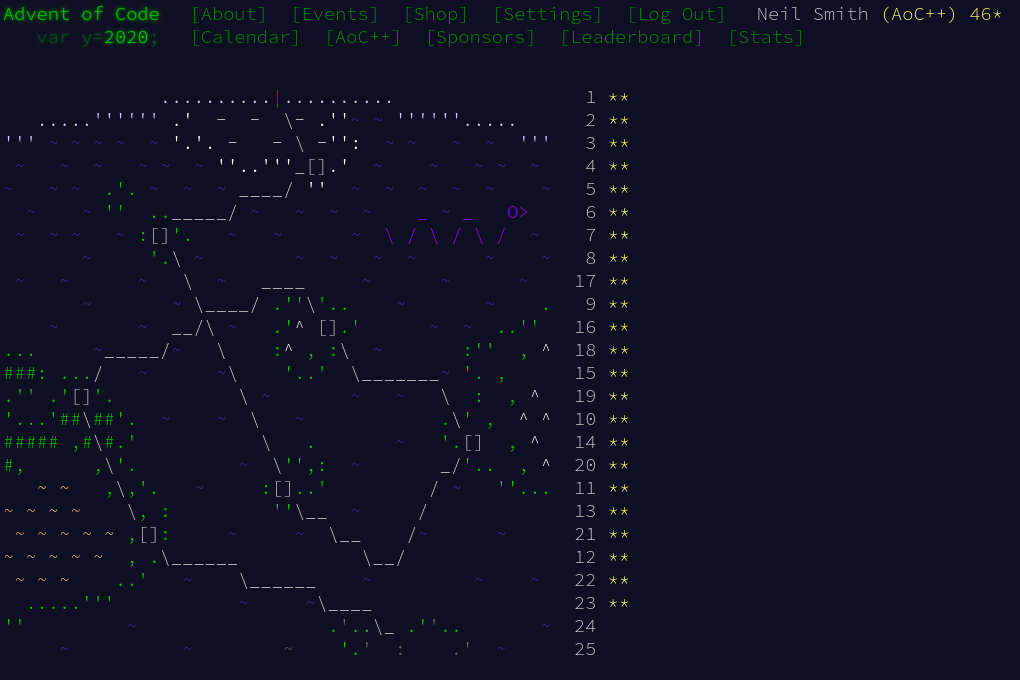January 8, 2021

# Advent of Code 2020 day 23

Starting simple, then changing direction for performanceDay 23 is one where the the obvious approach works fine for the small problem in part 1, but doesn't scale to the larger problem in part 2. But I think it's interesting to cover both, so this is a post of two parts.

## Part 1

This uses an obvious representation for the circle of cups, a circular pointed list. This is an example of a zipper, a data structure with one element picked out as a focus. In this case, the focus of the pointed list is the current element. Functions like previous and next move the focus, and you can insert and delete elements from the list. This is a natural fit to the "circle of labelled things" in the problem.

I needed to remove and re-insert three items, so I quickly whipped up takeRight and dropRight functions to help that.

takeRight :: Int -> P.PointedList a -> [a]
takeRight 0 _ = []
takeRight n xs = (xs ^. P.focus):(takeRight (n - 1) $P.next xs) dropRight :: Int -> P.PointedList a -> Maybe (P.PointedList a) dropRight 0 xs = Just xs dropRight n xxs = case (P.deleteRight xxs) of Just xs -> dropRight (n - 1) xs Nothing -> Nothing  (Note the use of a lens ^. to get the focused element; see AoC 2019 day 23 for more use of lenses.) I also needed something to find valid destinations validDestination 0 max missing = validDestination max max missing validDestination n max missing | n elem missing = validDestination (n - 1) max missing | otherwise = n  The main "play one step of the game" function is a fairly direct translation of the steps into code, inserting deleting and shifting as needed. playOne cups = P.next replacedAtCurrent where current = cups ^. P.focus held = takeRight 3$ P.next cups
shorter = fromJust $dropRight 3$ P.next cups
destination = validDestination (current - 1) 9 held
shorterAtDestination = fromJust $P.find destination shorter replaced = foldr P.insertRight shorterAtDestination$ reverse held
replacedAtCurrent = fromJust $P.find current replaced  Finally, a bit of plumbing to connect the input to the solution part1 nums = label$ playN cups 100
where cups = fromJust $P.fromList nums label cups = concatMap show$ tail $takeRight (P.length cups) atOne where atOne = fromJust$ P.find 1 cups

playN cups n = (iterate playOne cups) !! n


## Part 2

This scaled up from ten cups and one hundred rounds to one million cups and ten million rounds. A pointed list wasn't going to cope. But as all the moves were "what's the next cup around the circle", a circular linked list would be enough, if I could make the pointer updates happen in a mutable structure (much like day 15). It's easier to work through the updates, and the intermediate pointers needed, with an example.

I start with the cups after the first move of the example. Given the current cup, I can pick the first held cup and the first cup after the held ones.

I can then splice out the held cups by making current point to after held. I then identify the destination cup (and the cup after the destination).

Then I do the other pointer updates. Destination now points to held, and the last held cup points to the cup after the destination.

If I neaten that up a bit, I show the result after the move, ready for current to move on one cup.

Now I know what I'm doing, I just need to implement it. First, I need to decide on a data structure. Each cup has an index and a pointer to the next cup, and that's all. That suggests using a Vector, where the contents of cups[i] is the next cup from cup i. The state at in the last diagram above would be like this:

Index 1 2 3 4 5 6 7 8 9
3 5 2 6 4 7 8 9 1

After cup 1 comes cup 3; after cup 2 comes cup 5; and so on.

I also saw a suggestion (from somewhere) that keeps things a bit neater. Vectors have zero-based indexing, but I want to use one-based indexing for the pointers. I can repurpose cups to be a pointer to the current cup.

Index 0 1 2 3 4 5 6 7 8 9
2 3 5 2 6 4 7 8 9 1

After all of that, the code for each game step update follows from the description.

gameStep :: Int -> V.MVector s Int -> ST s ()
gameStep maxCups cups =
do  current <- V.read cups 0

-- close the loop, removing the held cups
V.write cups current afterHeld

let destination =
validDestination (current - 1) maxCups [held1, held2, held3]

-- make the held come after the destination
V.write cups destination held1

-- make the end of the held point into the rest of the loop
V.write cups held3 afterDestination

-- and store it
V.write cups 0 nextCup
return ()


There's also a bit of setup to create the initial vector then run the game.

runGame :: [Int] -> Int -> Int -> [Int]
runGame seed cupsNeeded roundsNeeded =
runST $do cups <- seedGame seed cupsNeeded gameLoop roundsNeeded cupsNeeded cups mapM (V.read cups) [0..cupsNeeded] seedGame :: [Int] -> Int -> ST s (V.MVector s Int) seedGame seed cupsNeeded = do cups <- V.new (cupsNeeded + 1) let extended = seed ++ [10, 11..] forM_ [0..cupsNeeded]$ \i -> V.write cups i (i + 1)
forM_ (zip seed $tail extended)$ \(i, j) -> V.write cups i j
let end = if cupsNeeded > (length seed)
then cupsNeeded
else last seed